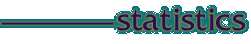Musical Influences Statistics In the attached pdf some additional statistics are offered in an effort to further summarize the influences data given in the "Composers" section on a composer-by-composer basis. In that section the relative removed age of influence is conveyed in the last two, "Influences", fields by preceding the names in each list with '+' and '-' signs. In each 'Musical Influences' field an individual's name is tagged as follows: (1) no tag: indicates that the influencing composer is older than the subject composer, and was alive during the latter's life; (2) one minus ( - ): indicates that the influencing composer died 0 to 25 years before the birth of the subject composer; (3) two minuses ( -- ): indicates that the influencing composer died 25 to 100 years before the birth of the subject composer; (4) three minuses ( --- ): indicates that the influencing composer died more than 100 years before the birth of the subject composer; (5) one plus ( + ): indicates that the influencing composer was younger than the subject composer. In each 'Has Influenced' field the reverse pattern is applied to identify relative temporal remoteness of influences generated *by* the subject composer. The first six columns in the pdf statistically summarize the influences *on* the subject composers. A mean temporal remoteness index ('Mean1') characterizing the influencing composers is calculated by assigning the values 0, -1, -2, -3, and +1 to the conditions described above, and then taking sums and means. For example, it turns out that the multiple composer influences on Carl Orff code temporally as: -3 0 -3 -3 -3 -3 0 0, which summed comes to a value of -15., which divided by the number of influences involved (8, as shown in the third column) produces a mean value of -1.88 (fifth column). The first column relays an unusual statistic: the number of composers influencing the subject composer who were *younger* than he/she was; the second column, the total number of minuses (for Orff, as above, 15). 'Mean2' (column four) for each subject composer is the mean of the index values associated with the individuals identified as influences on the subject (in the case of Orff, this is the mean of 6.9, 4.0, 2.6, 1.9, 2.1, 2.2, 3.7 and 3.8, or 3.40). Column six is the associated standard deviation (actually, the square root of the variance). The second group of six columns summarize each subject composers' influence on *other* composers. The first two of these parallel the first two in the first group, now totalling the number of composers influenced who are *older* than the subject ('Sum-'), and all the plusses connected with the composers influenced ('Sum+'; in the case of J. S. Bach this is a remarkable 274, a testimony to the extent of his lasting influence). The third column ('n/n+') gives the number of composers influenced by the subject, the first value being the raw total and the second, when present, a total weighted by instances of major influence. Column four ('Sum') for each subject composer is the mean of the index values associated with the individuals identified as influenced *by* the subject (in the case of Orff, this is 1.90, as he influenced only one other person, whose index value is 1.9). Column six is again the associated standard deviation; for Orff this is 0. because of the n of 1. The final column gives the overall rank of each composer in the list, for comparative purposes. Hyphens are placed in the table when no values can be calculated because no influences have been identified. retrieve PDF file here